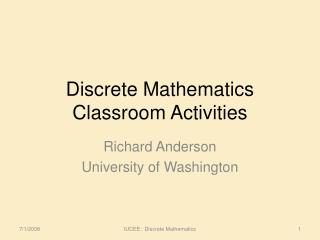DownloadDownload PresentationDiscrete Mathematics Classroom Activities

# Discrete Mathematics Classroom Activities

Download Presentation## Discrete Mathematics Classroom Activities

- - - - - - - - - - - - - - - - - - - - - - - - - - - E N D - - - - - - - - - - - - - - - - - - - - - - - - - - -
##### Presentation Transcript

1. Discrete MathematicsClassroom Activities Richard Anderson University of Washington IUCEE: Discrete Mathematics

2. Draw a picture of yourself

3. Inclusive or exclusive? • Coffee or tea comes with dinner • A password must have at least three digits or be at least eight characters long. • To take discrete mathematics, you must have taken calculus or a course in computer science. • You can pay using US Dollars or Indian Rupees. e, I, I, either

4. Rewrite in the form “if p then q” in English: It is necessary to walk 8 miles to get to the top of Long’s Peak. IUCEE: Discrete Mathematics

5. Translate into logic Do not use uniqueness operator Everyone has exactly one best friend IUCEE: Discrete Mathematics

6. Translate into logic There is some restaurant that serves some food that everyone likes IUCEE: Discrete Mathematics

7. Identify the domain, codomain, range, image of a, and the preimage of 2 A B 1 a b 2 c 3 d 4 e

8. How many zeros are there at the end of 100!

9. If a club has 25 members how many ways are there to choose the president, secretary, and treasurer, where no person can hold more than one office?

10. Suppose that E and F are events such that p(E) = 0.7 and p(F) = 0.5. Show that p(E  F)≥0.7 and P(E  F)≥0.2

11. Is R reflexive, symmetric, antisymmetric, transitive, if • R = {(x,y) | xy ≥ 1} • R = {(x,y) | x and y are both negative or both nonnegative} • R = {(x,y) | x ≥ y2}

12. What is the contrapositive of “if all cycles of G have even length, then G is bipartite”

13. Identify the strongly connected components of the graph

14. Draw a graph that has degree sequence 1, 2, 3, 3, 3

15. Draw a graph that has degree sequence 3, 3, 3, 3, 3

16. How many edges does Mn,m have?

17. Is the following pair of graphs isomorphic?